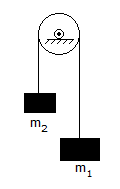# Civil Engineering - UPSC Civil Service Exam Questions

46.

On which one of the following concepts is the basic principle of structural design based ?

 A. Weak column strong beam B. Strong column and weak beam C. Equally strong column-beam D. Partial weak column-beam

Explanation:

In tall buildings, it is important to control lateral displacements within the serviceability limit state. A structural system may be classified as follows:

(i) Buildings frame system
(ii) Moment resisting frame system
(iii) Dual frame system
(iv) Tube system

In a moment resistant frame, the relative stiffness of beam and column is very important. A frame may be designed using weak column-strong beam proportions or strong column – weak beam proportions. A frame with weak beam - strong column is more stable and therefore highly desirable.

47.

Consider the following statements :
If two planes at right angles carry only shear stress of magnitude 'q', then the
1. diameter of Mohr's circle would equal 2q
2. centre of the Mohr's circle would lie at the origin
3. principal stresses are unlike and have magnitude 'q'
4. angle between the principal plane and the plane of maximum shear would be equal to 45° Of these statements :

 A. 1 and 2 are correct B. 2 and 4 are correct C. 3 and 4 are correct D. 1, 2, 3 and 4 are correct

Explanation:

No answer description available for this question. Let us discuss.

48.

Two bodies of masses m1 and m2 are communicated by a light inextensible string passing over a small smooth fixed pulley; m1 > m2. What is the acceleration of the system ?A. g (m1 + m2)/(m1 - m2) B. g (2m1 + m2)/(m1 - 2m2) C. g (m1 + 2m2)/(2m1 - m2) D. g (m1 - m2)/(m1 + m2)

Explanation:

No answer description available for this question. Let us discuss.

49.

Consider the following statements :
1. Butterfly valve is used for throttling and controlling flow rate.
2. Check valve is used in the discharge piping of a pump.
3. Air-relief valve is placed in the pipeline at the valleys to automatically vent the accumulated air in the system
4. Altitude valve is provided in user overhead tank.
Which of the statements given above are correct ?

 A. 1, 2 and 4 only B. 1, 2 and 3 only C. 1 and 2 only D. 3 and 4 only

Explanation:

No answer description available for this question. Let us discuss.

50.

A beam of rectangular section 100 mm x 300 mm carries certain loads such that the Bending Moment at a section A is M ans at another section B it is (M + C).The distance between the sections A and B is 0.5 m and there are no external loads acting between the two sections. If the value of C is 10, 000 Nm, then the maximum shear stress is

 A. 1.5 MN/m2 B. 1.0 MN/m2 C. 0.5 MN/m2 D. 0.25 MN/m2